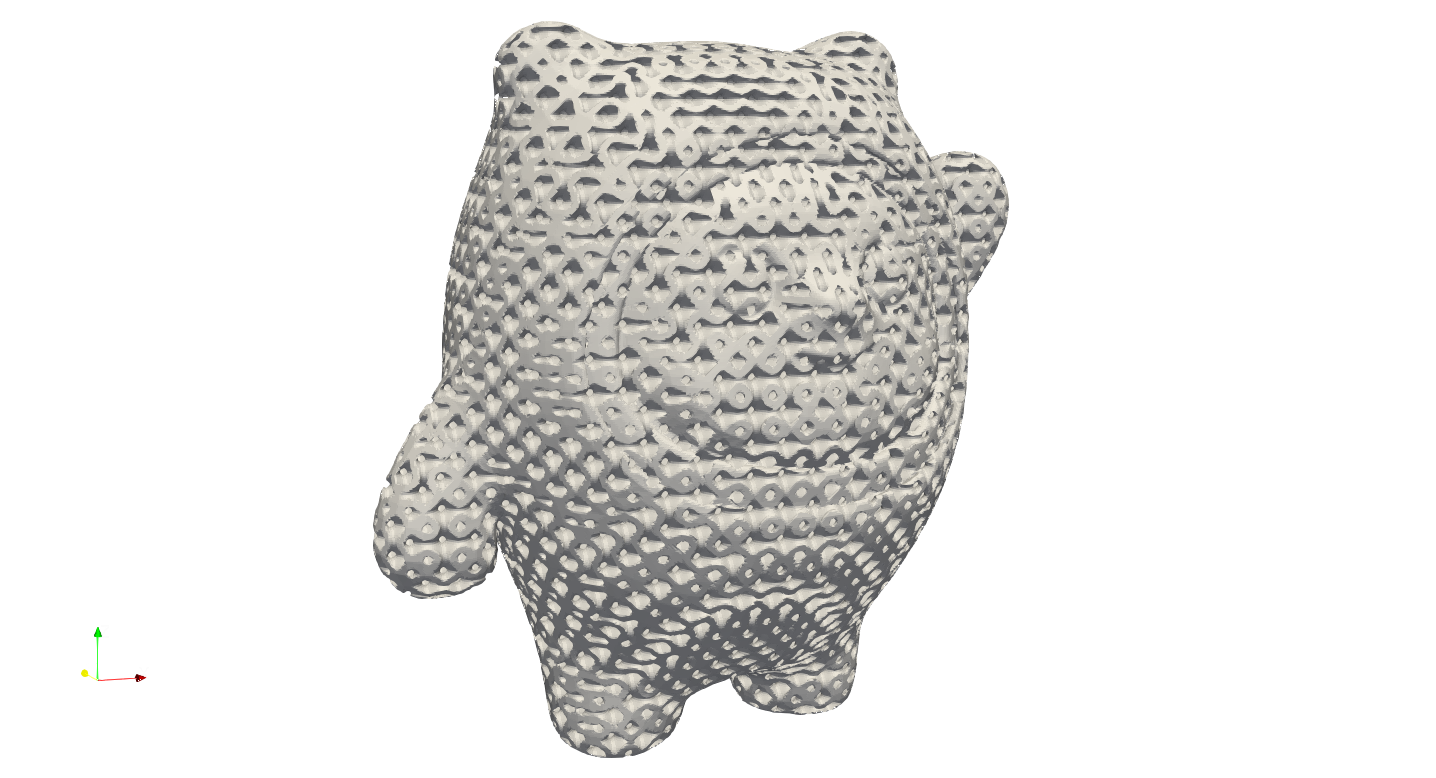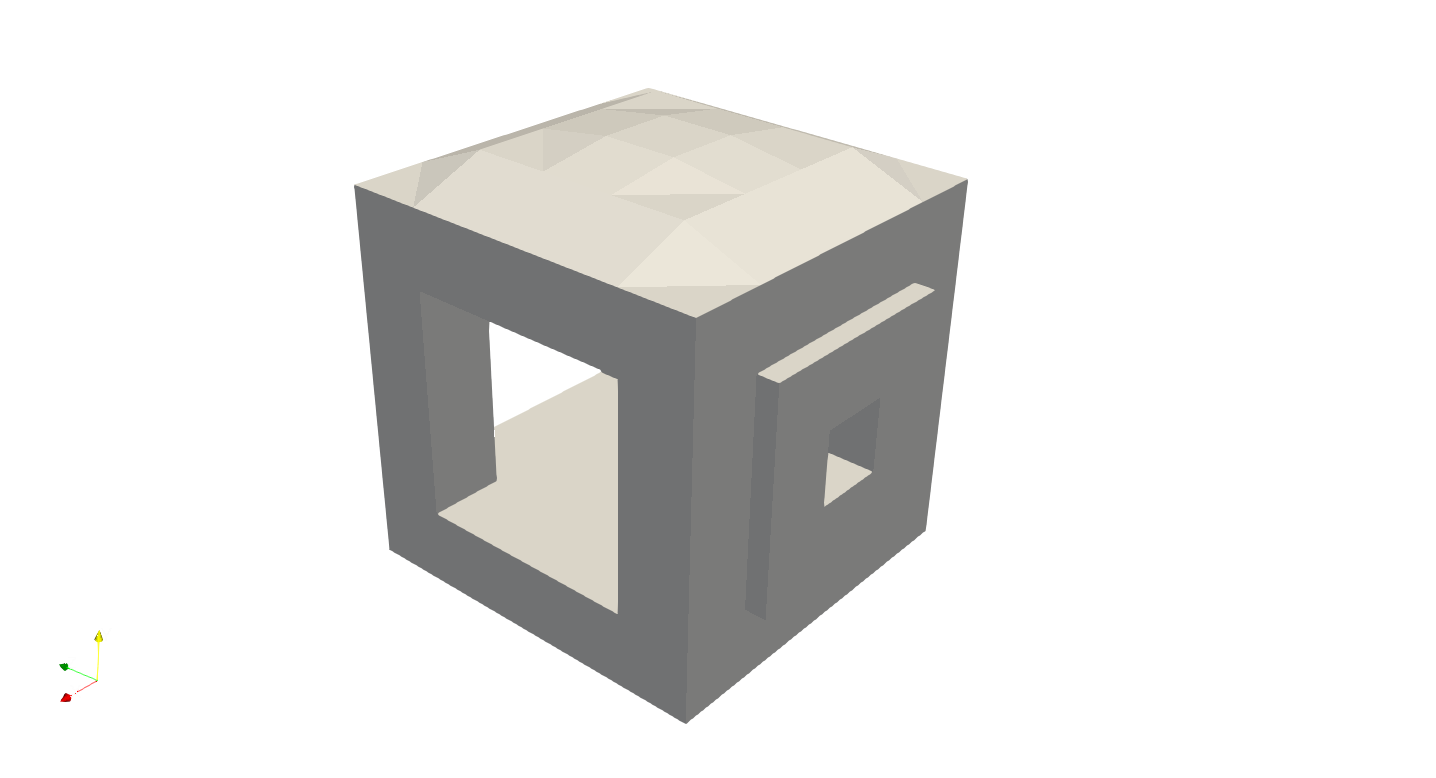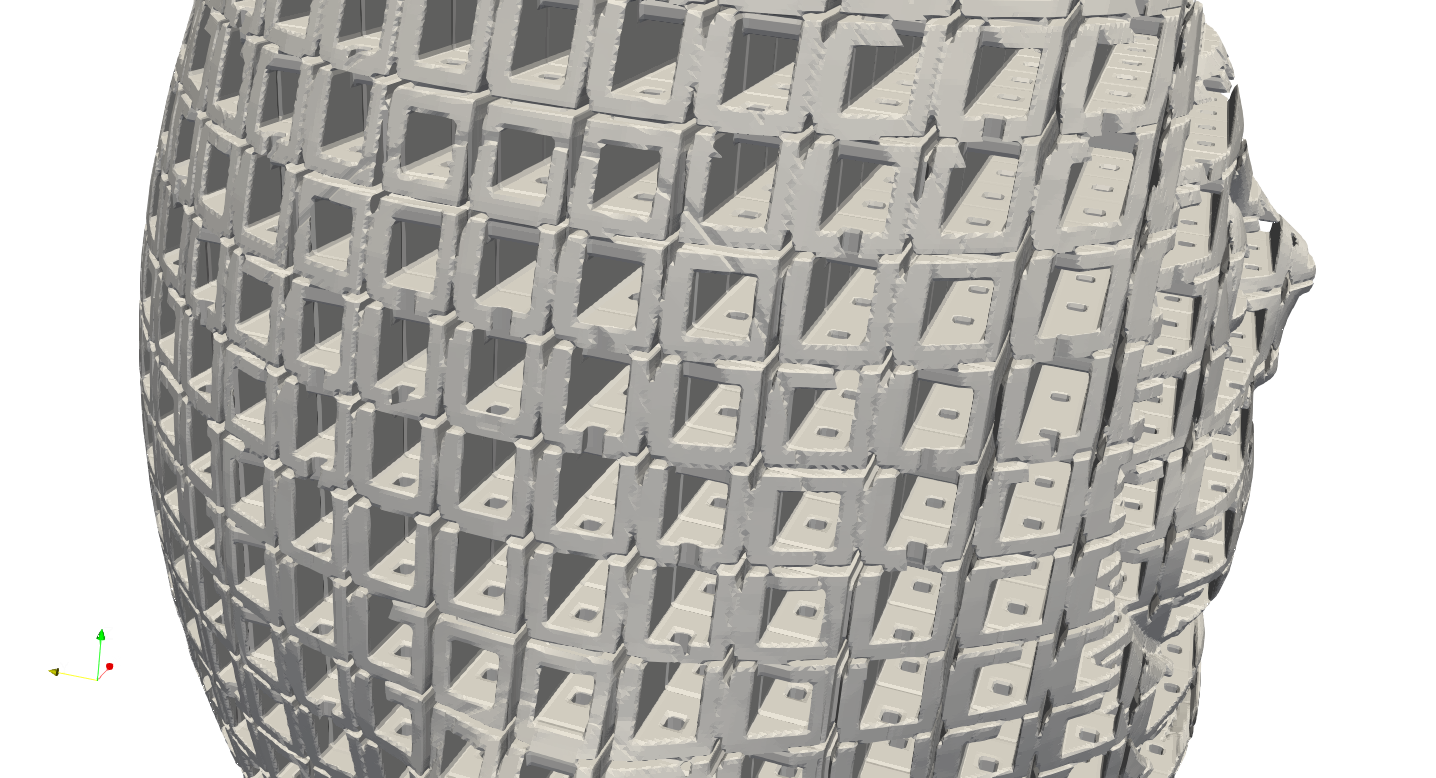# Customization#

Artisan integrates a number of lattice design, including:

Strut Lattice

TPMS Lattice

Strut Lattice Tetrahedron domain

Cubic, BCCubic, VertexOcta, BC, FCCubic, EdgeOcta, StarTet, Dodecahedron, Auxetic

SchwarzDiamond, SchwarzPrimitive, FischerKoch, Neovius, Lidinoid, Gyroid

Rhombic, Star, Icosahedral, Voronoi, Kagome, Tetrahedron

We will gradually expand this list above in future development. The strut and TPMS lattice generally used in hexahedron domain which contains 6 faces and 8 nodes, such as a cube. The strut lattice in tetrahedron domain is mainly used in conformal lattice generation when tetrahedron meshes were given. Artisan will not check the lattice domain types in filling process, but please note that, the mismatched lattices types will produce unexpected results. The lattice in tetrahedron domain will be demonstrated in later section.

This may not meet all user’s needs at some stage. Artisan has a capability of taking user’s lattice definition into the design. User may define a lattice through three different ways:

• Strut lattice, i.e. topological connections;

• Surface lattice, i.e. mathematical/implicit equations such as TPMS;

• Geometric shape, i.e. a file contains geometry mesh.

In practice, user only need to define la_name parameters in all keywords to the file of definitions. Please note this file has to be with extension of txt. Artisan automatically detects the customized definition and then interprets the content. The definition file contains a JSON structure that defines the type of customized lattice, and how the lattice defined.

Here we presented a few examples with keywords Add_Lattice, user may extend this feature to Lin_Interpolate, HS_Interpolate and Attractor keywords.

## Strut Lattice#

Defining a strut lattice requires the nodal coordinates, and topological conductivities, e.g. connection between two nodes. We use a simple geometry fill to demonstrate this functionality. Below is main example JSON which calls the user defined lattice.

{"Setup":{  "Type" : "Geometry",
"Geomfile": ".//sample-obj//shell_1_of_bdd_.stl",
"Rot" : [0.0,0.0,0.0],
"res":[0.5,0.5,0.5],
"onGPU": false,
"memorylimit": 16106127360
},
"WorkFlow":{
"la_name": ".//Test_json//CustomLattice_Strut.txt", "size": [8.0,8.0,8.0], "thk":1.2, "Rot":[0.0, 0.0, 0.0], "Trans":[0.0, 0.0, 0.0],
"Inv": false, "Fill": true, "Cube_Request": {}
}
},
"2":{"Export": {"outfile": ".//Test_results/BingDunDun_Infill_CustomStrut.stl"}}
},
"PostProcess":{"CombineMeshes": true,
"RemovePartitionMeshFile": false,
"RemoveIsolatedParts": true,
"ExportLazPts": false}
}


The parameter la_name in the keywords Add_Lattice became a file path that links to the custom strut lattice definition. The definition in the file is showed below. In this case, we defines the strut lattice type, and nodes coordinates and the conductivities. Note that the node coordinates shall define with from 0 to 1 mm in x, y and z direction. The parameter "ladomain" defines the lattice in a hexahedron lattice domain, i.e. "Hex", a domain defined by 6 faces and 8 nodes.

{
"type": "strut",
"definition": {
"pts":
[[1.0, 1.0, 0.0],
[1.0, 1.0, 1.0],
[0.0, 1.0, 1.0],
[0.0, 1.0, 0.0],
[0.0, 0.0, 0.0],
[0.0, 0.0, 1.0],
[1.0, 0.0, 1.0],
[1.0, 0.0, 0.0]],
"cnnt":[[0, 1],[0, 3],
[3, 2],[2, 1],
[1, 6],[0, 7],
[3, 4],[2, 5],
[7, 4],[7, 6],
[6, 5],[4, 5],
[0, 5],[1, 4],
[2, 7],[3, 6]],
}

}


Above example shall produce the filled results like below. Yes, it is filled by the BCCubic lattice. User may try different nodes locations and/or conductivities.## Surface Lattice#

User may define a surface through its implicit equation, and apply the thickness to the surface as a lattice unit. We can use a different user defined surface lattice.

{
"type": "TPMS",
"definition": {
"unit_x_len": "2*pi",
"unit_y_len": "2*pi",
"unit_z_len": "2*pi",
"expr": "cos(x)+cos(y)+cos(z)",
}


In this definition, we defined an implicit equation

$\cos(x) + \cos(y) + \cos(z) = 0$

We also need to tells Artisan the single unit length of the given euqation, here it is 2*pi meaning $$2\pi$$ . Artisan uses numexpr package to interpret the mathematical equations and inputs. User may refer to the documentation for referencing the acceptable math symbols and equations.

We can then have a TPMS style infill. Please note that, the size and thk in the keywords Add_Lattice will automatically define the unit lattice size and thicks the surface with correct thickness.## Geometry Lattice#

Artisan also supports user defined geometry infill. It meant the infill can be a geometry shape that made from other software. For example, we would like to put the following geometric shape as infill.To fill the geometry with this shape, we could define it like below.

{
"type": "Geom",
"definition": {
"file": ".//sample-obj//boxframe.obj",The thickness of the geometric shape lattice is adjustable through defining the parameter thk. The initial inputs will be considered as 0.0, the negative value of thk shrinks the shape, whereas the positive value expands the lattice unit. The thickness of the given shape has different definition in conformal lattice, please refer to conformal lattice section for more details.